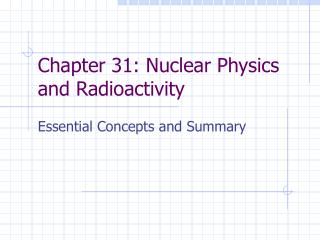Download PresentationChapter 31: Nuclear Physics and Radioactivity

# Chapter 31: Nuclear Physics and Radioactivity - PowerPoint PPT PresentationDownload Presentation## Chapter 31: Nuclear Physics and Radioactivity

- - - - - - - - - - - - - - - - - - - - - - - - - - - E N D - - - - - - - - - - - - - - - - - - - - - - - - - - -
##### Presentation Transcript

1. Chapter 31: Nuclear Physics and Radioactivity Essential Concepts and Summary

2. Nuclear Structure • Nucleus consists of neutrons and protons, called nucleons. • Atomic number = Z = number of protons in atom. • Atomic mass number = A = protons + neutrons • Isotopes: atoms with same number of protons, different number of neutrons

3. Strong Nuclear Force and Stability of Nucleus • Strong nuclear force holds nucleons together • As nucleii increase in size, they become less stable • Spontaneous disintegration or rearrangement of internal structure is called radioactivity

4. Mass Defect and Nuclear Binding Energy • Binding energy: Energy required to break apart nucleus • Sum of individual masses of nucleons is greater than sum of single nucleus. Difference in mass is known as mass defect

5. Radioactivity • Three kinds of rays are produced naturally: alpha, beta, and gamma • Alpha rays are helium nuclei • Beta rays are electron streams • Gamma rays are electromagnetic waves • Transmutation: process by which parent nuclei changes into different daughter nuclei via radioactive decay

6. Radioactive Decay and Activity • Half life: Time in which ½ of radioactive nuclei disintegrate • Activity: number of disintegrations per second • Lambda is proportionality constant known as decay constant • Amount left after time t related to initial amount and an exponential in t

7. Equation Summary